# 豹星

Leopardstar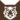虎族 (Q645)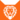狮族 (Q646)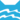河族 武士河族 副族长
（继任自橡心 (Q3239)河族 族长
（继任自钩星 (Q2630)虎族 族长
（继任自虎星 (Q712)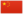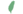小豹 Leopardkit  小豹
 豹爪 Leopardpaw  豹掌
 豹毛 Leopardfur  豹毛
 豹星 Leopardstar  豹星

Q2491：河族母猫，自《预言开始》系列登场的角色

## 细节

### 外貌

• 她是一只皮毛光滑、:23琥珀色眼睛:13的金色:猫物表斑点:303 虎斑母猫。:猫物表她身上有特别的:猫物表:1章金色斑点，:猫物表尾巴长而弯曲。:183

### 趣闻

• 她和蛾翅的毛色极为相似。:1章
• 她在五个月大时就成为了学徒，但这违背了武士守则:2章
• 她最喜欢的猎物是鲤鱼carp）:2章
• 她曾是一名干爪drypaw），这意味着她是不喜欢游泳的河族猫。:2章
• 她曾想要养育幼崽，但最终从未生育。:1章

## 语录

—— 豹星[注 1]放逐灰条，《险路惊魂》，第196-197页

—— 豹星拒绝派遣河族武士参加寻水远征队，《第四学徒》，第96-97页

—— 豹星对雾脚，在干旱后，《不为人知的故事》，第100页

—— 豹星向雾脚道歉，《雾星的征兆》，第1章

—— 豹星向雾脚表达悔意，《豹星的荣耀》英文版，第529-531页

—— 火星悼念豹星的死亡，《战声渐近》，第109页

## 登场书目

• 寒冰烈火 (Q59)[配角][首次登场🐱]
• 疑云重重 (Q60)[配角]
• 风起云涌 (Q65)[配角]
• 险路惊魂 (Q66)[配角]
• 力挽狂澜 (Q67)[配角]
• 午夜追踪 (Q139)[配角]
• 新月危机 (Q140)[对话提及]
• 重现家园 (Q141)[配角]
• 星光指路 (Q142)[配角]
• 黄昏战争 (Q143)[配角]
• 日落和平 (Q144)[配角]
• 预视力量 (Q152)[配角]
• 暗河汹涌 (Q153)[配角]
• 驱逐之战 (Q154)[猫物闲角]
• 天蚀遮月 (Q155)[配角]
• 暗夜长影 (Q156)[猫物闲角]
• 拂晓之光 (Q157)[配角]
• 第四学徒 (Q158)[配角]
• 战声渐近 (Q159)[对话提及][死亡时刻✝]
• 月光印记 (Q161)[对话提及]
• 群星之战 (Q163)[对话提及]
• 学徒探索 (Q170)[配角]
• 迷雾明光 (Q4621)[对话提及]
• 河翻浪涌 (Q4643)[配角]
• 火星的探索 (Q176)[配角]
• 钩星的承诺 (Q179)[配角]
• 灰条的誓言 (Q4057)[配角]
• 豹星的荣耀 (Q4426)[主人公][主要角色][视角人物]
• 一星的告解 (Q4826)[配角]
• 冬青叶的故事 (Q187)[猫物闲角]
• 雾星的征兆 (Q188)[配角][死亡时刻✝]
• 虎掌的愤怒 (Q190)[猫物闲角]
• 叶池的祈愿 (Q191)[猫物闲角]
• 红尾的恩债 (Q3565)[猫物闲角]
• 蛾翅的秘密 (Q3628)[配角]
• 黑脚的清算 (Q4336)[配角]
• 河族阴云 (Q4311)[配角]
• 变革之风 (Q4411)[配角]
• 返回族群 (Q212)[配角]
• 族群的秘密 (Q199)[追溯修订]
• 族群的猫 (Q200)[配角]
• 族群的战争 (Q202)[配角]
• 终极指南 (Q204)[配角]
• 族群决定 (Q4580)[配角]
• 超越守则：光灵的恻隐之心 (Q4321)[配角]
• ## 外部链接

### 官方文章

• 团结则存，分裂则亡 （隐射）
• 只是故事而已 （隐射）
• 原谅与改正 （提及）
• 沉重的要求 （提及）
• 角色聚光灯－豹星 （专题）
• 比憎恨更强大 （提及）
• 谨言慎行…… （提及）
• ## 脚注

1. 正体中文版中错写称“豹毛”。

## 参考文献

这篇文章基于CC BY-SA 3.0许可使用了猫武士维基（英语）Leopardstar一文中的部分内容。